Main Module 1 Presenting, Describing and Inferring from a Sample of Numerical Data

 Learning Objectives At the end of the module, the student will: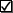Enter and analyze numerical data using Microsoft ExcelPresent a picture of the shape, center and variability of numerical dataPresent and interpret selected numerical measures of center, spread and shape of numerical dataUnderstand appropriate application of measures of center and spreadUse measures of center and spread to interpret the "Voice of the Process" and distinguish "noise" from process "signals" or outliersUnderstand process capability with respect to customer specification and process variability (compute probabilities)Use appropriate sample statistics to estimate a population meanConstruct and test hypothesis statements about a population mean
 Module Notes The following sub modules contain summary notes for the five topic areas of Module 1.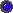Module 1.1: Why Statistics for Management?Module 1.2: Describing Data with PicturesModule 1.3: Describing Data with Numerical SummariesModule 1.4: Estimating with ConfidenceModule 1.5: Hypothesis-Testing Assignment Select a numerical variable, such as time to complete an activity, days to pay accounts or dollars of profit contribution. Gather 50 observations (you may adjust this up or down depending on data availability and the nature of the variable) and using Microsoft Excel: 1.  Enter the data. 2.  Sort data from lowest to highest values. 3. Create a histogram. 4.  Report descriptive statistics to include mean, standard deviation, first quartile, median, third quartile, and minimum and maximum values. 5.  Standardize the data. 6.  Remove outliers, if any, and re-compute descriptive statistics (Item 4). 7. Compute the probability of exceeding the 5th from last observation in the sorted data set. 8. Create a confidence interval for the population mean, using data with outliers (if any) removed. 9. Select a value for a true population mean for which you reject the null hypothesis. The hypothesis test may be one or two-tailed. Using an alpha of 0.05, carry out the hypothesis test in Excel. 10. Select a value for a true population mean for which you do not reject the null hypothesis. The hypothesis test may be one or two-tailed. Using an alpha of 0.05, carry out the hypothesis test in Excel. 11. Be able to demonstrate your knowledge of the learning objectives, as applied to this Assignment, in Exam 1. 12. (Optional) Send Microsoft Excel file, with items 1 - 10, as an attachment in an e-mail, to the instructor if you wish the instructor to review/give feedback on your work. This should be done not later than September 20, 2001 if you wish to receive instructor feedback. You may send parts of the assignment (such as Items 1 - 7) as you finish them, if you wish. 13. Notify instructor, via e-mail, when you have completed Assignment 1, and wish to take Exam 1. Include Assignment 1 as an attachment to your e-mail if you did not do Item 11, above. This needs to be done BEFORE September 22, 2001. E-mail or fax completed Exam 1 back to the instructor NOT LATER THAN September 22, 2001. Optional Text Reading Anderson, D., Sweeney, D., & Williams, T. (2001). Contemporary Business Statistics with Microsoft Excel. Cincinnati, OH: South-Western. Chapter 1 Chapter 2 (Pay particular attention to the Histogram) Chapter 3 (Skip Section 3.5) Chapter 6 (Section 6.2)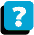About the Course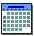Module Schedule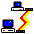WebBoard